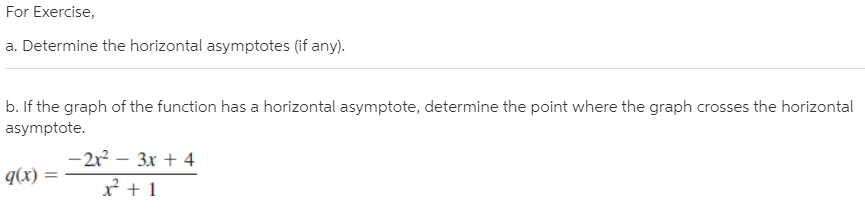# For Exercise, a. Determine the horizontal asymptotes (if any). b. If the graph of the function has a horizontal asymptote, determine the point where the graph crosses the horizontal asymptote. -2r – 3x + 4 |q(x) = r +1

Questionhelp_outlineImage TranscriptioncloseFor Exercise, a. Determine the horizontal asymptotes (if any). b. If the graph of the function has a horizontal asymptote, determine the point where the graph crosses the horizontal asymptote. -2r – 3x + 4 |q(x) = r +1 fullscreen